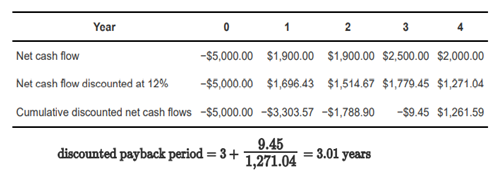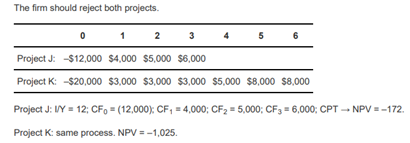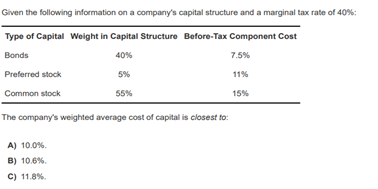# Buổi 12 - Corporate Finance - Reading 32: Capital Budgeting & Reading 33: Cost of Capital

READING 32: CAPITAL BUDGETING

LOS 32.a: Describe the capital budgeting process and distinguish among the various categories of capital projects

Question 32.1: Two of the typical steps in the capital budgeting process are:
A) analyzing project proposals and raising additional capital.
B) raising additional capital and creating the firm-wide capital budget.
C) analyzing project proposals and creating the firm-wide capital budget.
Explanation
C is correct. The four typical steps in the capital budgeting process are generating ideas, analyzing project proposals, creating the firm-wide capital budget, and monitoring decisions and conducting a post-audit. Raising additional capital is not one of the typical steps in the capital budgeting process.

Question 32.3: Which of the following is least relevant in determining project cash flow for a capital investment?
A) Sunk costs.
B) Tax impacts.
C) Opportunity costs
Explanation
A is correct. Sunk costs are not to be included in investment analysis. Opportunity costs and the project's impact on taxes are relevant variables in determining project cash flow for a capital investment.
Schweser note
Sunk costs are costs that cannot be avoided, even if the project is not undertaken. Because these costs are not affected by the accept/reject decision, they should not be included in the analysis. An example of a sunk cost is a consulting fee paid to a marketing research firm to estimate demand for a new product prior to a decision on the project.

LOS 32.d: Calculate and interpret net present value (NPV), internal rate of return (IRR), payback period, discounted payback period, and profitability index (PI) of a single capital project.

Question 32.4: An analyst identifies the following cash flows for an average-risk project:
Year 0       −\$5,000
Year 1-2      \$1,900
Year 3         \$2,500
Year 4         \$2,000
If the company's cost of capital is 12%, the project's discounted payback period is closest to:
A) 2.5 years.
B) 3.0 years.
C) 3.9 years
Explanation
B is correct.Question 32.5: Wreathfield, Inc., is choosing between two mutually exclusive projects. The cash flows for the two projects are below. The firm has a cost of capital of 12%, and the risk of the projects is equivalent to the average risk of the firm.
0           1         2            3            4            5           6
Project J:   –12,000    4,000    5,000    6,000
Project K:   –20,000   3,000    3,000    3,000     5,000    8,000     8,000
Wreathfield should accept:
A) Project J.
B) Project K.
C) Neither project J nor project K.
Explanation
C is correct.LOS 32.e: Explain the NPV profile, compare the NPV and IRR methods when evaluating independent and mutually exclusive projects, and describe the problems associated with each of the evaluation methods.

Question 32.6: Inverness Corporation is considering investing in one of two mutually exclusive projects. The firm's cost of capital is 15%. Project One's NPV profile crosses the vertical axis at \$1.8 million and crosses the horizontal axis at 25%. Project Two's NPV profile crosses the vertical axis at \$1.2 million and crosses the horizontal axis at 33%. For the two projects, the crossover rate is 18%. Inverness should:
A) accept Project One.
B) accept Project Two.
C) reject both projects.
Explanation
A is correct. The NPV of Project One is higher than the NPV of Project Two at any discount rate less than the crossover rate, and the NPVs of both projects are positive at the firm's cost of capital. Because Project One and Project Two are mutually exclusive, the firm should select Project One because it has the higher NPV at a discount rate of 15%.

Question 32.7: Which of the following is least likely a problem associated with the internal rate of return (IRR) method of choosing investment projects?
A) Using IRR to rank mutually exclusive projects assumes reinvestment of cash flows at the IRR.
B) For independent projects, the IRR and NPV can lead to different investment decisions.
C) If the project has an unconventional cash flow pattern, the result can be multiple IRRs.
Explanation
B is correct. IRR and NPV lead to the same decision when choosing independent projects but may lead to different decisions when choosing between projects.

LOS 32.f: Contrast the NPV decision rule to the IRR decision rule and identify problems associated with the IRR rule.

Question 32.8: If a project has a negative cash flow during its life or at the end of its life, the project most likely has:
A) more than one internal rate of return.
B) a negative internal rate of return.
C) multiple net present values.
Explanation
A is correct. Projects with unconventional cash flows (where the sign of the cash flow changes from minus to plus to back to minus) will have multiple internal rates of return. However, one will still be able to calculate a single net present value for the cash flow pattern.
Schweser note
If a project has cash outflows during its life or at the end of its life in addition to its initial cash outflow, the project is said to have an unconventional cash flow pattern. Projects with such cash flows may have more than one IRR (there may be more than one discount rate that will produce an NPV equal to zero).

LOS 32.g: Describe expected relations among an investment’s NPV, company value, and share price
Question 32.9: A company announces a new project that an analyst believes will require an investment of \$5 per share this year and will increase aftertax cash flow by \$1 per share in each of the next eight years. Assuming this project is new information and does not have any signaling effects, what should the analyst estimate as the impact of acceptance of the project on the value of the company's shares?
A) An increase of \$3.
B) Either an increase or a decrease.
C) An increase of less than \$3.
Explanation

B is correct. Other things equal, the new project should change the share value by the net present value (NPV) of the new project per share. Although the project has a positive internal rate of return (IRR) (total inflows are greater than the initial outlay), no information is given about the company's cost of capital. If the company's cost of capital is less than the project's IRR, the project's NPV will be positive and share value will be increased. If the cost of capital is greater than the project's IRR, the project will have a negative NPV and decrease share value.

READING 33: COST OF CAPITAL

LOS 33.a: Calculate and interpret the weighted average cost of capital (WACC) of a company.

Question 33.1:Explanation
B is correct.

LOS 33.b: Describe how taxes affect the cost of capital from different capital sources.

Question 33.2: Over the next year, Thatherton Co. is expecting their marginal tax rate to increase by 5%. Also, over the next 12 months, Thatherton plans to undertake several expansion projects significantly more risky than previous projects. Thatherton Co.'s current capital structure includes 40% debt and 60% equity. Which of the following statements correctly summarizes the effect these changes will have on the company's marginal cost of capital?
A) The increasing tax rate will increase the MCC.
B) The riskier projects will increase the MCC.
C) Both the increasing tax rate and the riskier projects will increase the MCC.
Explanation
B is correct. Recall that the marginal cost of capital (MCC) is the cost of the last dollar of new capital raised by the firm. Marginal cost increases as increasing amounts of capital are raised during a set period. In general, firms in riskier businesses, or with riskier projects, have higher costs of common equity and thus higher MCC and WACC. The increase in the tax rate would reduce the after-tax cost of debt, reducing the MCC.

Question 32.3: An analyst is studying the tax exposures and capital structures of Alpha Corporation and Beta Corporation. Both companies have equivalent weights of debt and equity in their capital structures. Pretax component costs of capital are the same for both companies. Alpha has total capital of \$850 million while Beta has total capital of \$370 million. The marginal tax rates for Alpha and Beta are 35% and 40%, respectively. Which of the following statements regarding Alpha and Beta is least accurate?
A) Beta Corporation has a lower WACC than Alpha Corporation.
B) An increase in Alpha Corporation’s tax rate would decrease its WACC.
C) A tax rate change will affect Alpha Corporation’s cost of equity more than Beta Corporation.
Explanation
C is correct.

LOS 33.c: Describe the use of target capital structure in estimating WACC and how target capital structure weights may be determined

Question 32.4: Which of the following choices best describes the role of taxes on the after-tax cost of capital in the U.S. from the different capital sources?
Common equity       Preferred equity        Debt
A)           Decrease               Decrease              No effect
B)           No effect                No effect               Decrease
C)           No effect                Decrease              Decrease
Explanation
B is correct. In the U.S., interest paid on corporate debt is tax deductible, so the after-tax cost of debt capital is less than the before-tax cost of debt capital. Dividend payments are not tax deductible, so taxes do not decrease the cost of common or preferred equity.

LOS 33.d: Explain how the marginal cost of capital and the investment opportunity schedule are used to determine the optimal capital budget.

Question 32.5: A firm's optimal capital budget can be found by moving along its investment opportunity schedule until:
A) it exhausts its capital budget.
B) average project return is equal to average cost of capital.
C) the next project’s return is less than the marginal cost of capital.
Explanation
C is correct. The firm would not want to exhaust its capital budget on "bad" projects, (i.e., projects with IRR < cost of capital [NPV < 0]). They should continue to invest as long as the project's return is greater than the marginal cost of capital of the firm. When the project's IRR = cost of capital, the NPV = 0. Such a project will only make the firm larger; it will add nothing to the stock value. The investment opportunity schedule plots expected project returns from highest to lowest IRR.
Schweser note
The intersection of the investment opportunity schedule with the marginal cost of capital curve identifies the amount of the optimal capital budget. The intuition here is that the firm should undertake all those projects with IRRs greater than the cost of funds, the same criterion developed in the capital budgeting topic review. This will maximize the value created. At the same time, no projects with IRRs less than the marginal cost of the additional capital required to fund them should be undertaken, as they will erode the value created by the firm

LOS 33.e: Explain the marginal cost of capital’s role in determining the net present value of a project.

Question 32.6: Which of the following statements about the role of the marginal cost of capital in determining the net present value of a project is most accurate? The marginal cost of capital should be used to discount the cash flows:
A) for potential projects that have a level of risk near that of the firm’s average project.
B) of all projects the firm is considering.
C) if the firm’s capital structure is expected to change during the project’s life.
Explanation
A is correct. Net present values of projects with the average risk for the firm should be determined using the firm's marginal cost of capital. The discount rate should be adjusted for projects with above-average or belowaverage risk. Using the marginal cost of capital assumes the firm's capital structure does not change over the life of the project.

LOS 33.g: Calculate and interpret the cost of noncallable, nonconvertible preferred stock

Question 33.7: The Garden and Home Store recently issued preferred stock paying \$2 annual dividends. The price of its preferred stock is \$20. The after-tax cost of xed-rate debt capital is 6% and the cost of common stock equity is 12%. The cost of preferred stock is closest to:
A) 10%.
B) 9%.
C) 11%.
Explanation
A is correct. Preferred stock pays constant dividends into perpetuity. The price of preferred stock equals the present value of the preferred stock dividends: \$20 = \$2 / kps . Therefore, the cost of preferred stock capital equals \$2 / \$20 = 0.10 = 10%
Schweser note
The cost of preferred stock (kps) is:
kps = Dps/ P
where:
Dps = preferred dividends
P = market price of preferred

LOS 33.h: Calculate and interpret the cost of equity capital using the capital asset pricing model approach, the dividend discount model approach, and the bond-yieldplus risk-premium approach.

Question 33.8: Faye Harlan, CFA, is estimating the cost of common equity for Cyrene Corporation. She prepares the following data for Cyrene:
Price per share = \$50.
Expected dividend per share = \$3.
Expected retention ratio = 30%.
Expected return on equity = 20%.
Beta = 0.89.
Yield to maturity on outstanding debt = 10%.
The expected market rate of return is 12% and the risk-free rate is 3%. Based on these data, Harlan determines that Cyrene's cost of common equity is 14%. Harlan most likely arrived at this estimate by using the:
A) dividend discount model approach.
B) capital asset pricing model approach.
C) bond yield plus risk premium approach.
Explanation
C is corect.
Step 1: Calculate cost of common equity using the CAPM approach.
The estimated cost of common equity = 3% + 0.89 (12% − 3%) = 11%.
Step 2: calculate cost of common equity using the dividend discount model approach, the growth rate = (0.3)(0.2) = 6% and the estimated cost of common equity = \$3 / \$50 + 6% = 12%.
Step 3: Conclude
To get a cost of common equity of 14%, Harlan most likely added a risk premium to Cyrene's bond yield.

LOS 33.i: Calculate and interpret the beta and cost of capital for a project

Question 33.9: Jay Construction Company is considering whether to accept a new bridge-building project. Jay will use the pure-play method to estimate the cost of capital for the project, using Cass Bridge Builders as a comparable company. To calculate the project beta, Jay should:
A) estimate Cass’s cost of equity capital and apply it to the project.
B) use the CAPM equation, substituting Cass’s equity beta for its own.
C) adjust Cass’s equity beta for any difference in leverage between Cass and Jay.
Explanation
C is correct. To use the pure-play method, an asset beta is calculated by removing the effects of leverage (delevering) from the comparable company's equity beta, then a project beta is estimated by adjusting the asset beta (relevering) based on the capital structure of the company that is evaluating the project.
Schweser note
Because a specific project is not represented by a publicly traded security, we typically cannot estimate a project’s beta directly. One process that can be used is based on the equity beta of a publicly traded firm that is engaged in a business similar to, and with risk similar to, the project under consideration. This is referred to as the pure-play method because we begin with the beta of a company or group of companies that are purely engaged in a business similar to that of the project and are therefore comparable to the project. Thus, using the beta of a conglomerate that is engaged in the same business as the project would be inappropriate because its beta depends on its many different lines of business

LOS 33.j: Describe uses of country risk premiums in estimating the cost of equity.

Question 33.10: One of the primary limitations of using beta in calculating the cost of equity in a developing country is:
A) the market portfolio in developing countries is often not well diversied.
B) beta does not capture country risk.
C) beta does not capture inflation risk.
Explanation
B is correct. Because beta does not capture country risk, we add a country risk premium to the market risk premium when calculating expected returns using the CAPM.

LOS 33.k: Describe the marginal cost of capital schedule, explain why it may be upward-sloping with respect to additional capital, and calculate and interpret its breakpoints.

Question 33.11: A company's schedule of the costs of debt and equity shows that an additional \$3 million of debt can be issued at an after-tax cost of 3%, and additional equity of \$9 million can be issued at a cost of 6%. The company plans to maintain a capital structure of 30% debt and 70% equity. At what level of new capital financing will the marginal cost of capital change with the issuance of new debt?
A) \$3 million.
B) \$10 million.
C) \$13 million.
Explanation
B is correct. The break point at which new debt will increase the marginal cost of capital is calculated as \$3 million (the level of debt when the cost will change) divided by the percent of debt in the capital structure (30%). Break point = \$3 million / 0.3 = \$10 million in total new capital.
Schweser note
Break points occur any time the cost of one of the components of the company’s WACC
changes. A break point is calculated as:
Break point = amount of capital at which the component's cost of capital changes/weight of the component in the structure